# Python set with examples

This article provides you with an easy way to learn all about "sets" in Python with the help of not only theory but also example programs and their respective outputs. This article deals with:

## Set definition in Python

Set, like List, Tuple, and Dictionary, uses a single variable to store one or more elements (of any data type).In other words, we can say that a set is a data type that can be used to store collections of data or multiple items.

## Set syntax in Python

Set items must be written in curly braces, as shown in the sample code below:

`marks = {89, 87, 92, 67}`

### Precautions for Using Curly Braces

If you use curly braces to initialize an empty set, then it gets treated as a dictionary. Therefore, use the set() constructor to do the job. Here is an example:

```my_set_one = {}
print(type(my_set_one))
my_set_two = set()
print(type(my_set_two))```

Here is its sample output: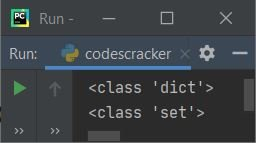Note: The type() method returns the type of the variable passed as its argument.

### Set items are unordered

Unlike List, Tuple, and Dictionary, Set items are unordered. That is, if you print the set, then you'll get all the items in the set, but in a random order, as shown in the sample output produced by the program given below:

```marks = {89, 87, 92, 67, 78, 94}
print(marks)```

Here is its sample output: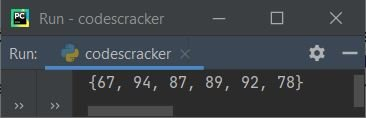As you can see, the original order of the set is 89, 87, 92, 67, 78, and 94, but the output's order is 67, 94, 87, 89, 92, and 78.

### Set items can not be indexed

Set items, unlike lists and tuples, cannot be accessed via their indexes.Therefore, sets in Python do not support any type of slicing operation.

### Set items are unchangeable

Like tuples, set items are also unchangeable. That is, after initializing the set items, we cannot change the item.

### Set items do not allow duplicate values

Set, like Dictionary, does not allow duplicate values. The program given below demonstrates it:

```marks = {89, 87, 94, 87}
tmarks = marks
print(tmarks)```

Here is its sample output: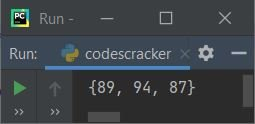As you can see, the mark 87 appears twice in the same set; therefore, one 87 gets removed automatically.

### Using Set, extract unique elements from a list

Since sets do not allow duplicates, we can use sets to extract all the unique elements from a list, as done in the program given below:

```places = ["NewYork", "California", "NewYork", "Los Angles", "California"]
unique_places = set(places)
print("\nUnique Places are:", unique_places)```

Here is its sample output:To remove all duplicates and return another list, use the following code:

`list(set(places))`

In the above code, the list named "places" gets converted into a set. As a result, the set removes duplicates and is converted back into a list.So the variable places are now a list of only unique elements.

## Adding new items to a set in Python

It is possible to add new items to a set. The program given below illustrates how to add new items to an already created set.

```marks = {89, 87, 92, 67, 78, 94}
print(marks)
print(marks)```

The snapshot given below shows the output produced by the above Python program: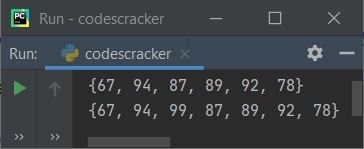## The set() constructor in Python

In place of curly braces, the set() constructor can also be used to define a set, as shown in the program given below:

```marks = set((89, 87, 94))
print("My Marks are:", marks)```

The image below shows an example of the output this Python program produces: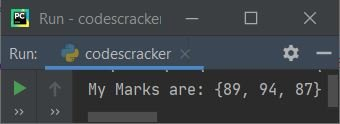Important: Remember the double (()) or brackets.

## Set operations in Python

With the help of sets in Python, we can perform:

• union using "|"
• intersection using "&"
• difference using "-"
• symmetric difference using "^"

of any two sets, just as in mathematics. The following program illustrates and demonstrates all four well-known set operations:

```set_one = {1, 2, 3, 4, 5}
set_two = {2, 5, 7, 8, 9}
print("\n------------Union of Two Sets------------------------")
print(set_one, "|", set_two, "=", set_one | set_two)
print("\n------------Intersection of Two Sets-----------------")
print(set_one, "&", set_two, "=", set_one & set_two)
print("\n------------Difference of Two Sets-------------------")
print(set_one, "-", set_two, "=", set_one - set_two)
print("\n------------Symmetric Difference of Two Sets---------")
print(set_one, "^", set_two, "=", set_one ^ set_two)```

This program produces the output that looks like: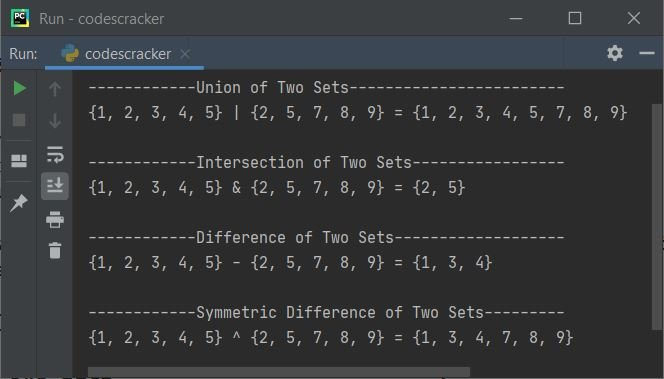The other two famous operations on the sets are checking "issubset" and "issuperset." The table given below shows methods and their equivalent operators used to perform operations on sets, supposing a and b are two sets:

Method Operator Method Example Operator Example
intersection() & a.intersection(b) a & b
union() | a.union(b) a|b
difference() - a.difference(b) a - b
symmetric_difference() ^ a.symmetric_difference(b) a ^ b
issubset() <= a.issubset(b) a <= b
issuperset() >= a.issuperset(b) a >= b

## Python Set Example

Here is an example of sets in Python:

```mysets = {1, 2, (3, 4, 5), 6, (7, 8), (9, 10)}
print(mysets)```

Here is its sample output: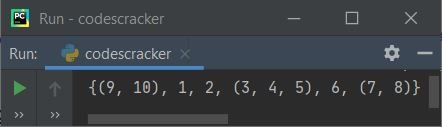#### Set example with items of multiple data types

The example program given below on sets shows a set with items of multiple data types that are of string, boolean, and int types:

```student_detail = {"codescracker", True, 100}
print(student_detail)```

Here is its sample output: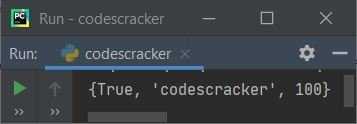#### Add multiple elements to a set

Now the program given below shows how to add multiple elements to a set. The user must enter the element's size and the element itself at run-time, as shown in the program and sample run below:

```myset = {1, 2, 3}

print("-------Original Set-------")
print(myset)

print("\nHow many elements to add in the set ?")
tot = int(input())
print("Enter", tot, "elements:")
for i in range(tot):
val = input()

print("\n--------New Set-----------")
print(myset)```

Here is its sample output after providing exactly 4 as "How many elements to add in the set?" and then 100, 200, 300, and 400 as four elements to add: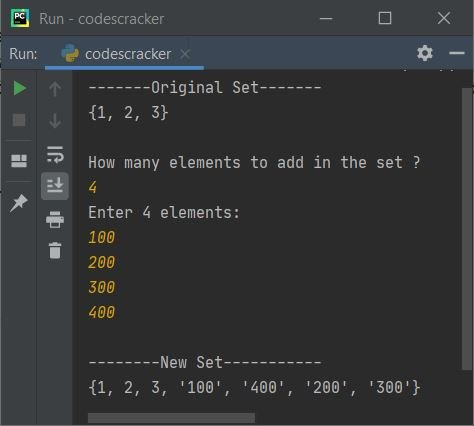Note: You're seeing the entered element added as a string data type because, while receiving the input using the input() method, we haven't used the int() method to convert the input value to an integer type. That is, anything received using input() gets treated as a string type by default.

## Add two sets in Python

Along with the addition of new items to the set, we can also add the whole set to another set. To do this job, the update() method helps to join two sets, as shown in the program given below:

```first_sem_marks = {89, 87, 94}
print(first_sem_marks)
second_sem_marks = {90, 93, 70}

first_sem_marks.update(second_sem_marks)
print(first_sem_marks)```

Here is its sample output:## Find the length of a set in Python

The len() method is available in Python to achieve the goal of finding and printing the length of a set. Here is an example program that illustrates it:

```student_detail = {"codescracker", "Programmer", True, 100}
print("Set Items:", student_detail)
print("Set Length:", len(student_detail))```

This program produces the output as shown in the snapshot given below:## Check if an element is available in SET or not

The program given below shows how to check whether an element is available in the set or not:

```myset = {1, 2, 3}

print("Enter an Element to check: ")
val = int(input())

if val in myset:
print(val, "is available in the Set")
else:
print(val, "is not available in the Set")```

Here is its sample run with user input of "3"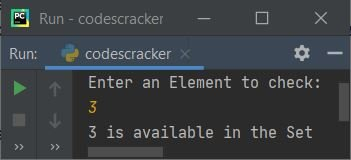## Remove an element from a set in Python

Python provides two methods to do the job of removing an element from a set. The two methods are remove() and discard(). The following program is an example of using both methods to remove an element from a set:

```myset = {1, 2, 3, 4, 5}
print("------Original Set------")
print(myset)

print("-----Set after removing 5------")
print(myset)

myset.remove(2)
print("-----Set after removing 2------")
print(myset)```

The snapshot given below shows the sample output produced by this program: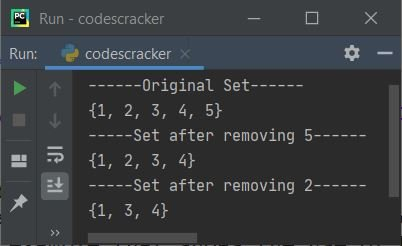To remove any random elements from the set, use the pop() method. And to remove all the items from a set, use the clear() method. The example program given below uses and illustrates these two methods:

```myset = {1, 2, 3, 4, 5}
print("------Original Set------")
print(myset)

myset.pop()
print("-----Set after popping------")
print(myset)

myset.clear()
print("-----Set after clearing-----")
print(myset)```

The output of this Python program is: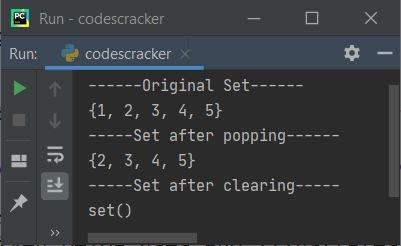Python Online Test

« Previous Tutorial Next Tutorial »